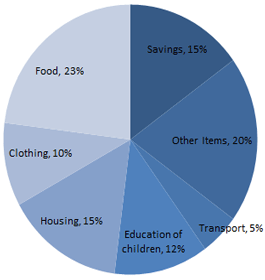The pie chart drawn belown shows the expenses of a family on various items and its savings during the year 2001. Study the graph and answer the question.(a) Maximum expenditure of the family was on :
[A]Education of child
[B]Housing
[C]Food
[D]Other items

Food
Maximum expenditure of the family is on food, which is 23%.

(b) The total savings of the family for the year were equal to the expenditure on :
[A]Food
[B]Housing
[C]Clothing
[D]Other items including transport

Housing
∵ Expenditure on housing = Savings = 15%.

(c) What per cent of income was spent on transport and other items together?
[A]20%
[B]25%
[C]32%
[D]30%

25%
% Expenditure on transport and other items = (20 + 5) % = 25%.

(d) If the total income of the family was 100000 Rs., how much money was spent on the education of the children?
[A]23000 Rs.
[B]15000 Rs.
[C]12000 Rs.
[D]10000 Rs.

12000 Rs.
Expenditure on the education of the children$= 12\% of 100000 Rs.$$= \frac{12\times 100000}{100}Rs.$$= 12000 Rs.$
Hence option [C] is the right answer.

(e) If the total income for the year was 100000 Rs. the difference of the expensses (in rupees) between housing and transport was:
[A]7000 Rs.
[B]10000 Rs.
[C]15000 Rs.
[D]12000 Rs.

10000 Rs.
The percentage difference in expenditure on housing and transport = (15 – 5) % = 10%
The required difference$= 10\% of 100000$$= 10000 Rs.$
Hence option [B] is the right answer.